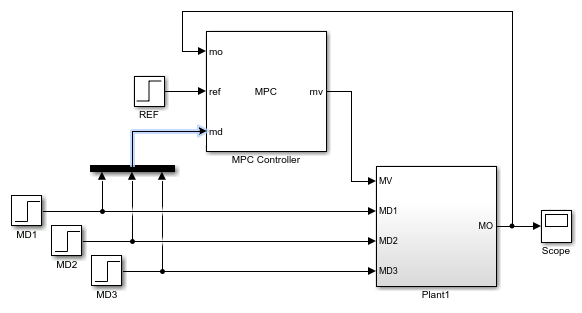Linearize Simulink Models Using MPC Designer

This topic shows how to linearize Simulink® models using MPC Designer. To do so, open the app from a Simulink model that contains an MPC Controller block. For this example, use the CSTR_ClosedLoop model.

sys = 'CSTR_ClosedLoop';
open_system(sys)

In the model window, double-click the MPC Controller block.

In the Block Parameters dialog box, ensure that the MPC Controller field is empty, and click to open MPC Designer.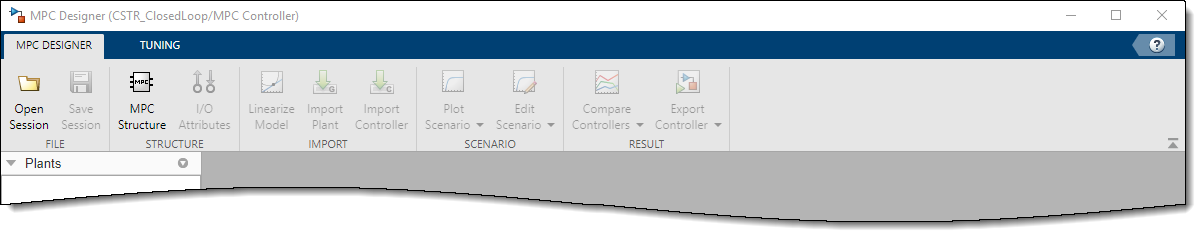Using MPC Designer, you can define the MPC structure by linearizing the Simulink model. After you define the initial MPC structure, you can also linearize the model at different operating points and import the linearized plants.

Note

If a controller from the MATLAB® workspace is specified in the MPC Controller field, the app imports the specified controller. In this case, the MPC structure is derived from the imported controller. In this case, you can still linearize the Simulink model and import the linearized plants.

Define MPC Structure By Linearization

This example shows how to define the plant input/output structure in MPC Designer by linearizing a Simulink model.

On the MPC Designer tab, in the Structure section, click MPC Structure.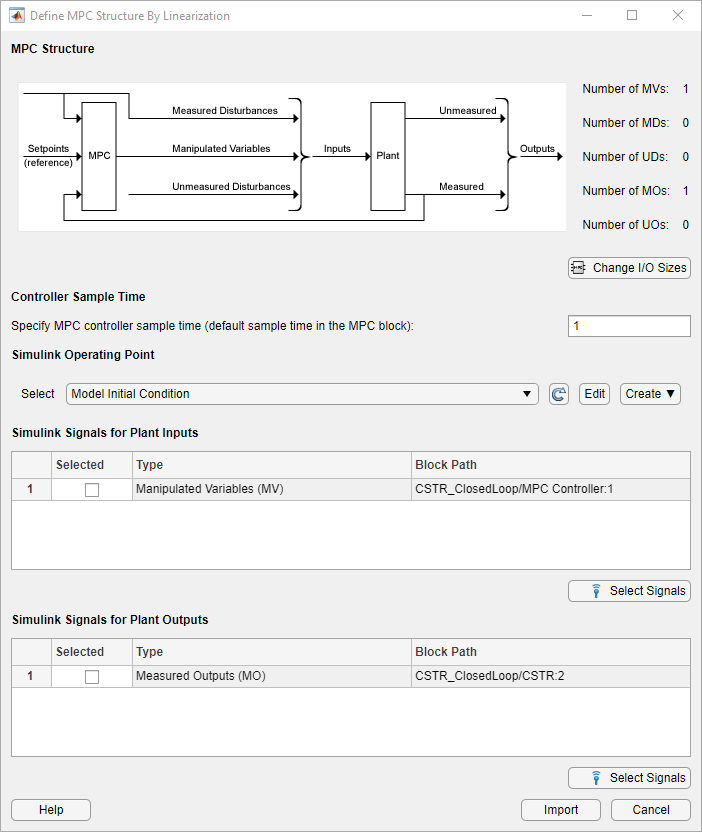Specify Signal Dimensions

In the Define MPC Structure By Linearization dialog box, in the MPC Structure section, if the displayed signal dimensions do not match your model, click to configure the dimensions. Any unmeasured disturbances or unmeasured outputs in your model are not detected by the MPC Controller block. Specify the dimensions for these signals.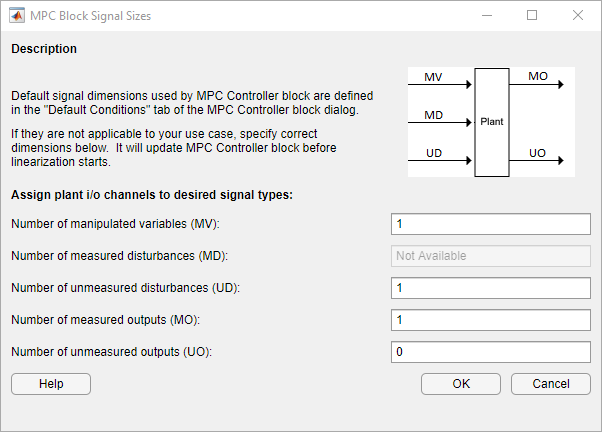Tip

In the MPC Controller Block Parameters dialog box, in the Default Conditions tab, you can define the controller sample time and signal dimensions before opening MPC Designer.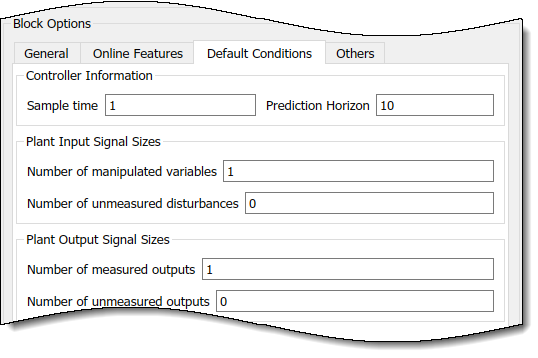Select Plant Input/Output Signals

Before linearizing the model, assign Simulink signal lines to each MPC signal type in your model. The app uses these signals as linearization inputs and outputs.

In the Simulink Signals for Plant Inputs and Simulink Signals for Plant Outputs sections, the Block Path is automatically defined for manipulated variables, measured outputs, and measured disturbances. MPC Designer detects these signals since they are connected to the MPC Controller block. If your application has unmeasured disturbances or unmeasured outputs, select their corresponding Simulink signal lines.

To choose a signal type, use the Selected option buttons.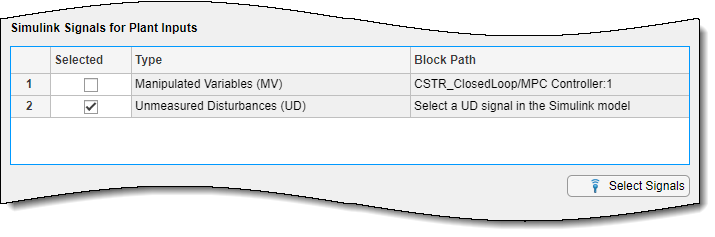Click .

In the Simulink model window, click the signal line corresponding to the selected signal type.

The signal is highlighted, and its block path is added to the Select signals dialog box.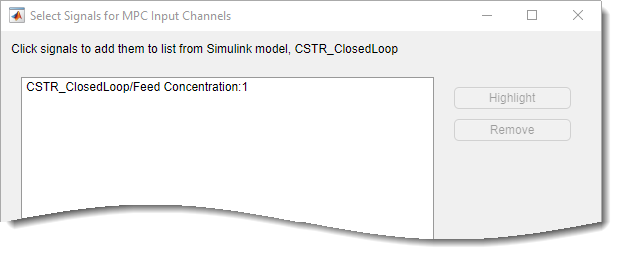In the Select signals dialog box, click .

In the Define MPC Structure By Linearization dialog box, the Block Path for the selected signal type updates.

Note

If your model has measured disturbances, you must connect the corresponding plant inputs to the signal line connected to the md port of the MPC Controller block. For more information, see Connect Measured Disturbances for Linearization.

Specify Operating Point

In the Simulink Operating Point section, in the drop-down list, select an operating point at which to linearize the model.

For information on the different operating point options, see Specifying Operating Points.

Note

If you select an option that generates multiple operating points for linearization, MPC Designer uses only the first operating point to define the plant structure and linearize the model.

Define Structure and Linearize Model

Click .

The app linearizes the Simulink model at the specified operating point using the specified input/output signals, and adds the linearized plant to the Data Browser.

Also, a default controller, which uses the linearized plant as its internal model, and a default simulation scenario are created.

MPC Designer uses the input/output signal values at the selected operating point as nominal values.

Linearize Model

After you define the initial MPC structure, you can linearize the Simulink model at different operating points and import the linearized plants. Doing so is useful for validating controller performance against modeling errors.

On the MPC Designer tab, in the Import section, click Linearize Model.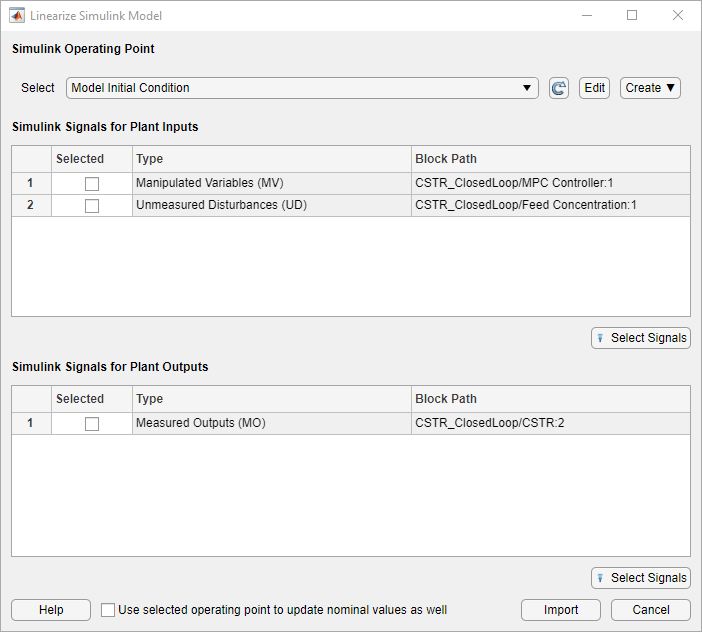Select Plant Input/Output Signals

In the Simulink Signals for Plant Inputs and Simulink Signals for Plant Outputs sections, the input/output signal configuration is the same as you specified when initially defining the MPC structure.

You cannot change the signal types and dimensions once the structure has been defined. However, for each signal type, you can select different signal lines from your Simulink model. The selected lines must have the same dimensions as defined in the current MPC structure.

Specify Operating Point

In the Simulink Operating Point section, in the drop-down list, select the operating points at which to linearize the model.

For information on the different operating point options, see Specifying Operating Points.

Linearize Model and Import Plant

Click .

MPC Designer linearizes the Simulink model at the defined operating point using the specified input/output signals, and adds the linearized plant to the Data Browser.

If you select the Use selected operating point to update nominal values as well option, the app updates the controller nominal values using the operating point signal values.

If you select an option that generates multiple operating points for linearization, the app linearizes the model at all the specified operating points. The linearized plants are added to the Data Browser in the same order in which their corresponding operating points are defined. If you choose to update the nominal values, the app uses the signal values from the first operating point.

Specifying Operating Points

In the Simulink Operating Point section, in the drop-down list, you can select or create operating points for model linearization. For more information on finding steady-state operating points, see About Operating Points (Simulink Control Design) and Compute Steady-State Operating Points from Specifications (Simulink Control Design).

When using MPC Designer in MATLAB Online™, you must linearize your model at the model initial conditions.

Select Model Initial Condition

To linearize the model using the initial conditions specified in the Simulink model as the operating point, select Model Initial Condition.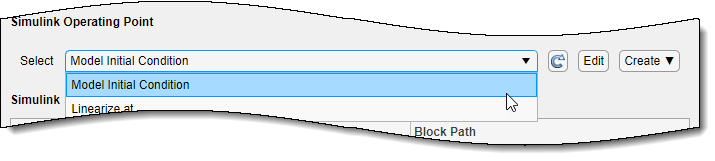The model initial condition is the default operating point for linearization in MPC Designer.

Linearize at Simulation Snapshot Times

To linearize the model at specified simulation snapshot times, select Linearize At. Linearizing at snapshot times is useful when you know that your model reaches an equilibrium state after a certain simulation time.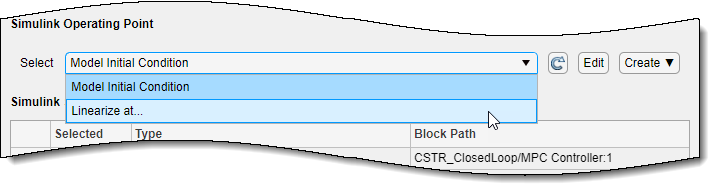In the Enter snapshot times to linearize dialog box, in the Simulation snapshot times field, enter one or more simulation snapshot times. Enter multiple snapshot times as a vector.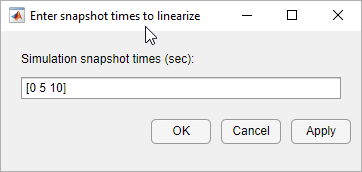Click .

If you enter multiple snapshot times, and you selected Linearize At from the:

• Define MPC Structure By Linearization dialog box, MPC Designer linearizes the model using only the first snapshot time. The nominal values of the MPC controller are defined using the input/output signal values for this snapshot.

• Linearize Simulink Model dialog box, MPC Designer linearizes the model at all the specified snapshot times. The linearized plant models are added to the Data Browser in the order specified in the snapshot time array. If you selected the Use selected operating point to update nominal values as well option, the nominal values are set using the input/output signal values from the first snapshot.

To compute a steady-state operating point using numerical optimization methods to meet your specifications, select Trim Model.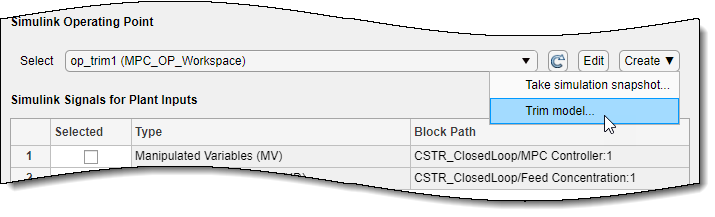In the Trim the model dialog box, enter the specifications for the steady-state values at which you want to find an operating point. You can specify values for states, input signals, and output signals.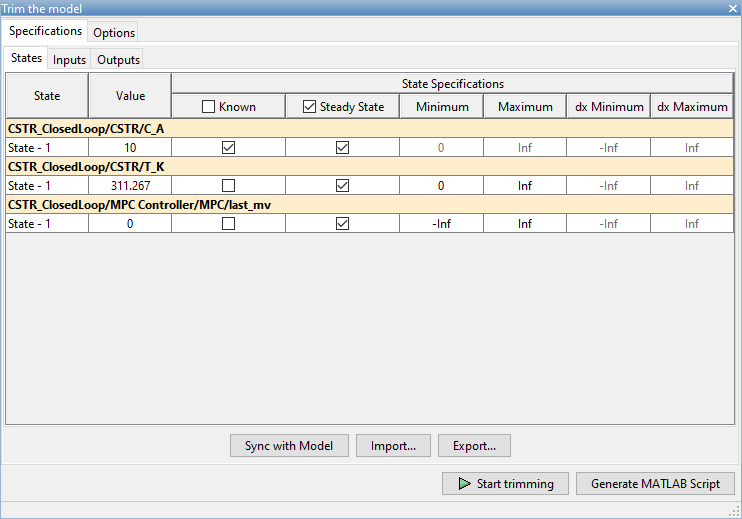Click Start Trimming.

MPC Designer creates an operating point for the given specifications. The computed operating point is added to the Simulink Operating Point drop-down list and is selected.

For examples showing how to specify the conditions for a steady-state operating point search, see Compute Steady-State Operating Points from Specifications (Simulink Control Design).

Compute Operating Point at Simulation Snapshot Time

To compute operating points using simulation snapshots, select Take Simulation Snapshot. Linearizing the model using operating points computed from simulation snapshots is useful when you know that your model reaches an equilibrium state after a certain simulation time.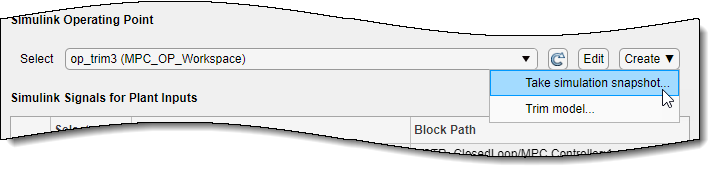In the Enter snapshot times to linearize dialog box, in the Simulation snapshot times field, enter one or more simulation snapshot times. Enter multiple snapshot times as a vector.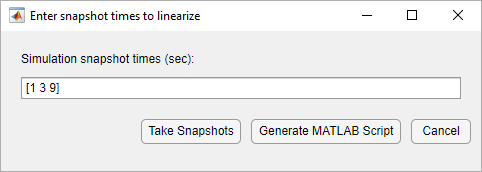Click .

MPC Designer simulates the Simulink model. At each snapshot time, the current state of the model is used to create an operating point, which is added to the drop-down list and selected.

If you entered multiple snapshot times, the operating points are stored together as an array. If you selected Take Simulation Snapshot from the:

• Define MPC Structure By Linearization dialog box, MPC Designer linearizes the model using only the first operating point in the array. The nominal values of the MPC controller are defined using the input/output signal values for this operating point.

• Linearize Simulink Model dialog box, MPC Designer linearizes the model at all the operating points in the array. The linearized plant models are added to the Data Browser in the same order as the operating point array.

In MPC Designer, the and Take Simulation Snapshot options generally produce the same linearized plant and nominal signal values. However, since the Take Simulation Snapshot option first computes an operating point from the snapshot before linearization, the results can differ.

Select Existing Operating Point

Under Existing Operating Points, select a previously defined operating point at which to linearize the Simulink model. This option is available if one or more previously created operating points are available in the drop-down list.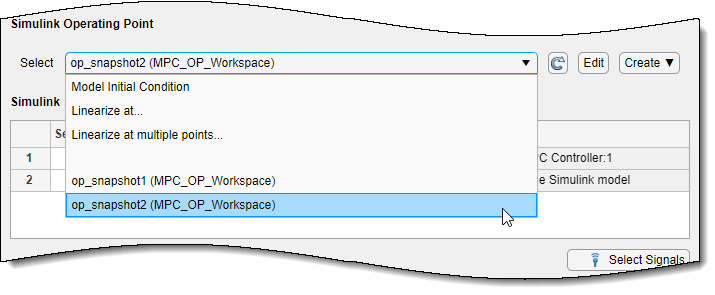If the selected operating point represents an operating point array created using multiple snapshot times, and you selected an operating point from the:

• Define MPC Structure By Linearization dialog box, MPC Designer linearizes the model using only the first operating point in the array. The nominal values of the MPC controller are defined using the input/output signal values for this operating point.

• Linearize Simulink Model dialog box, MPC Designer linearizes the model at all the operating points in the array. The linearized plant models are added to the Data Browser in the same order as the operating point array.

Select Multiple Operating Points

To linearize the Simulink model at multiple existing operating points, select Linearize at Multiple Points. This option is available if there are more than one previously created operating points in the drop-down list.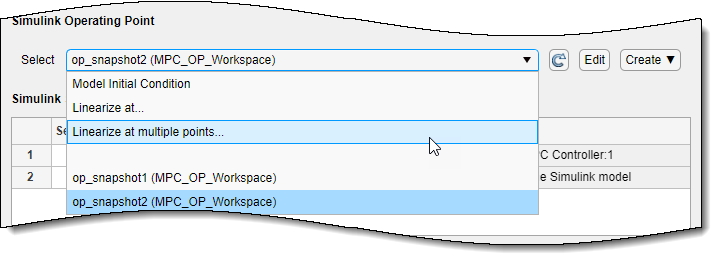In the Specify multiple operating points dialog box, select the operating points at which to linearize the model.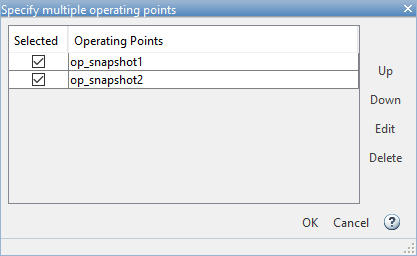To change the operating point order, click an operating point in the list and click or to move the highlighted operating point within the list.

Click .

If you selected Linearize at Multiple Points from the:

• Define MPC Structure By Linearization dialog box, MPC Designer linearizes the model using only the first specified operating point. The nominal values of the MPC controller are defined using the input/output signal values for this operating point.

• Linearize Simulink Model dialog box, MPC Designer linearizes the model at all the specified operating points. The linearized plant models are added to the Data Browser in the order specified in the Specify multiple operating points dialog box.

View/Edit Operating Point

To view or edit the selected operating point, under View/Edit, click the Edit option.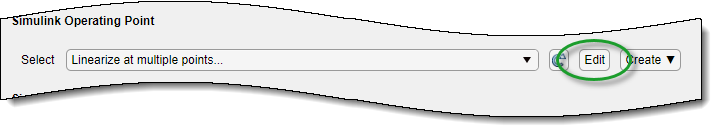In the Edit dialog box, if you created the selected operating point from a simulation snapshot, you can edit the operating point values.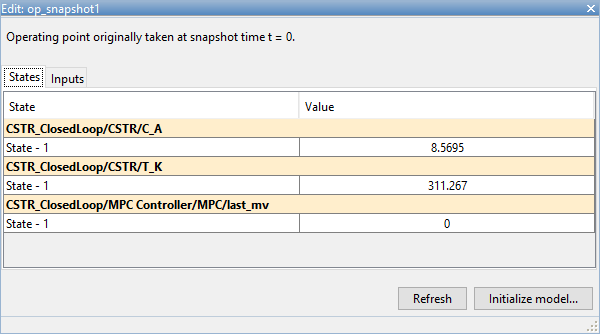If the selected operating point represents an operating point array, in the Select Operating Point drop-down list, select an operating point to view.

If you obtained the operating point by trimming the model, you can only view the operating point values.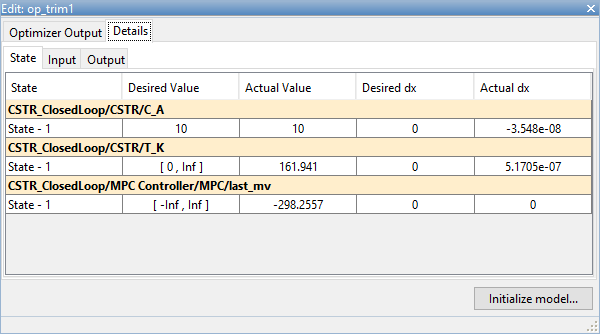To set the Simulink model initial conditions to the states in the operating point, click . You can then simulate the model at the specified operating point.

When setting the model initial conditions, MPC Designer exports the operating point to the MATLAB workspace. Also, in the Simulink Configuration Parameters dialog box, in the Data Import/Export section, it selects the Input and Initial state parameters and configures them to use the states and inputs in the exported operating point.

To reset the model initial conditions, for example if you delete the exported operating point, clear the Input and Initial state parameters.

Connect Measured Disturbances for Linearization

If your Simulink model has measured disturbance signals, connect them to the corresponding plant input ports and to the md port of the MPC Controller block. If you have multiple measured disturbances, connect them to the MPC Controller using a vector signal. As discussed in Define MPC Structure By Linearization, MPC Designer automatically detects the measured disturbances connected to the MPC Controller block and sets them as plant inputs for linearization.

Since the measured disturbances connected to the md port are selected as linearization inputs, you must connect the plant measured disturbance input ports to the selected signal line, as shown in the following:

Correct MD ConnectionIf you connect the plant measured disturbance input ports to the corresponding signals before the Mux block, as shown in the following, there is no linearization path from the signals at the md port to the plant. As a result, when you linearize the plant using MPC Designer, the measured disturbance channels linearize to zero.

Incorrect MD Connection